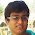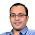## Oct 23, 2010

### Two creepers climbing a tree

Source: Asked to me by Nigel Coldwell (now posted on his blog)

Problem: Two creepers, one jasmine and other rose, are both climbing up and round a cylindrical tree trunk. jasmine twists clockwise and rose anticlockwise, both start at the same point on the ground. before they reach the first branch of the tree the jasmine had made 5 complete twists and the rose 3 twists. not counting the bottom and the top, how many times do they cross?

Related video:

Solution: My solution posted on Nigel's Blog here. Slightly different solution posted by Gaurav Sinha (1996 CSE IITK passout, now at Indian Revenue Service) in comments! A more general argument by Aaditya Ramdas (CMU Grad Student - CSE IITB 2009 Alumnus - Ex Tower Research Analyst) in comments!

1.should be 7.

If we take projection of their movement on floor in two dimensions, then they are moving in a circle. Jasmine does 5 clockwise circles and rose does 3 anti clockwise circles. If we define f and g be functions of time, s.t. f(t) is number of circles completed by jasmine and g(t) is number of circles by rose. f+g is a monotonically increasing conntinous function of time.

Then initially, f+g=0. At end, f+g=5+3=8.
They cross when f+g is integer i.e when f+g =1,2,3,4,5,6,7. As f+g is continous and increasing function, each of these values of f+g occurs exactly once.

2.Nice explanation. Thanks.

3.For (n,m)==(m,n), the answer should be m+n-1 - you can solve it using induction. Note that (0,1)~0. Assume (m,n)~m+n-1, we get (m+1,n)~m+n (by imagination? imagine (m,n), now add 1 loop above topmost intersection, you get exactly 1 more intersection).

This answer is also very easy to imagine independent of proof method. Imagine rose loops around m times and then goes straight up to the top, but jasmine goes straight up and then loops n times (imagine the figure with the rose loops below and jasmine loops above) - we trivially see m+n-1 intersections.

4.Clarifying Ramdas's comment:

Even if the creepers don't grow uniformly in perfect circles, if we know that they have completed m and n rounds, we can be sure that they intersected m+n-1 times in between.

This can be proved by induction.

Let f(m,n) be number of intersections for rose completing m rounds and jasmine completing n rounds.

TPT f(m,n) = m+n-1

Note that f(m,n)=f(n,m) by symmetry
Also note that f(0,1)=0.

Assume f(m,n)=m+n-1,
we get f(m+1,n)=m+n (by imagination? imagine f(m,n), now add 1 loop above topmost intersection, you get exactly 1 more intersection).

Since f(m,n)=f(n,m)
we get f(m,n+1)=m+n

Hence proved.

One very intuitive case:
Imagine rose loops around m times and then goes straight up to the top, but jasmine goes straight up and then loops n times (imagine the figure with the rose loops below and jasmine loops above) - we trivially see m+n-1 intersections.

5.Another way to see the solution is by "opening" the cylinder. Both the creepers are then traveling in straight lines (and in opposite direction) with breaks in a wrap-around way, when they complete the circumference of the trunk. Ratio of the magnitude of slope is 5:3. After drawing this figure, one can easily figure out that the number of intersections are indeed 7.

ps: my apologies for such a cryptic answer...would have been better if i cud use a figure.

6.@Vishal.. A friend gave me a similar solution 3 days back.

Basically, you want to open up your cylinder into a rectangle to width 2*pi*r and height h.

The image you are discussing can be found here. The circled points denote the 7 points of intersection.

7.Non mathematical Solution :
whenever they meet, their x,y,z, coordinates are same. Assuming their uniform speed (ans since they meet at the end at the same time)=> their speed in z-direction is same.
So, we can consider this in 2 dimensions as their z-coordinate isalways same.
One make 5 rounds => 10* pie
Other make 3 round => 6*pie.
So, relatively 16 * pie => 8 intersection => Ans is 7 as last one is not to be counted.

For general m and n rounds, It would give m+n-1 as answer.

1.Good solution. :-) Thanks

### Fraction Brainteaser

Source: Sent to me by Gaurav Sinha Problem: Siddhant writes a Maths test and correctly answers 5 out of 6 Arithmetic questions and 20...